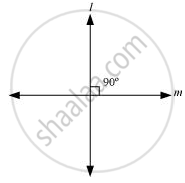# Give a definition for perpendicular lines. Are there other terms that need to be defined first? What are they, and how might you define them? - Mathematics

Give a definition for perpendicular lines. Are there other terms that need to be defined first? What are they, and how might you define them?

#### Solution

Perpendicular lines :-

If two lines intersect each other at 90°, then these are called perpendicular lines.

We are required to define line and the angle before defining perpendicular lines.Is there an error in this question or solution?
Chapter 5: Introduction to Euclid's Geometry - Exercise 5.1 [Page 85]

#### APPEARS IN

NCERT Class 9 Maths
Chapter 5 Introduction to Euclid's Geometry
Exercise 5.1 | Q 2.2 | Page 85

Share